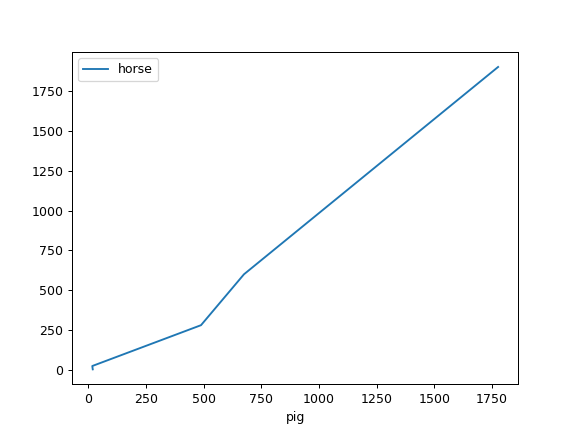# pandas.DataFrame.plot.line¶

`DataFrame.plot.``line`(x=None, y=None, **kwargs)[source]

Plot Series or DataFrame as lines.

This function is useful to plot lines using DataFrame’s values as coordinates.

Parameters
xlabel or position, optional

Allows plotting of one column versus another. If not specified, the index of the DataFrame is used.

ylabel or position, optional

Allows plotting of one column versus another. If not specified, all numerical columns are used.

colorstr, array_like, or dict, optional

The color for each of the DataFrame’s columns. Possible values are:

• A single color string referred to by name, RGB or RGBA code,

for instance ‘red’ or ‘#a98d19’.

• A sequence of color strings referred to by name, RGB or RGBA

code, which will be used for each column recursively. For instance [‘green’,’yellow’] each column’s line will be filled in green or yellow, alternatively.

• A dict of the form {column namecolor}, so that each column will be

colored accordingly. For example, if your columns are called a and b, then passing {‘a’: ‘green’, ‘b’: ‘red’} will color lines for column a in green and lines for column b in red.

New in version 1.1.0.

**kwargs

Additional keyword arguments are documented in `DataFrame.plot()`.

Returns
matplotlib.axes.Axes or np.ndarray of them

An ndarray is returned with one `matplotlib.axes.Axes` per column when `subplots=True`.

`matplotlib.pyplot.plot`

Plot y versus x as lines and/or markers.

Examples

```>>> s = pd.Series([1, 3, 2])
>>> s.plot.line()
```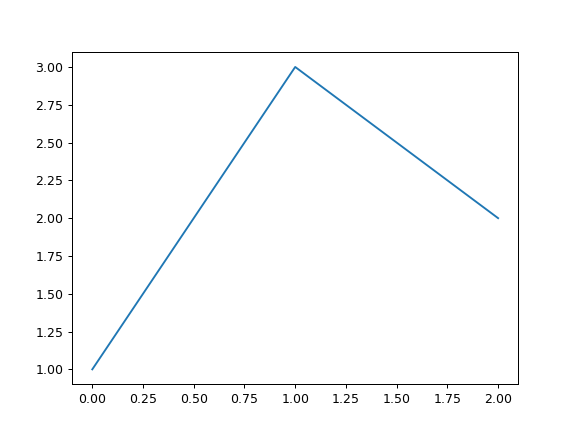The following example shows the populations for some animals over the years.

```>>> df = pd.DataFrame({
...    'pig': [20, 18, 489, 675, 1776],
...    'horse': [4, 25, 281, 600, 1900]
...    }, index=[1990, 1997, 2003, 2009, 2014])
>>> lines = df.plot.line()
```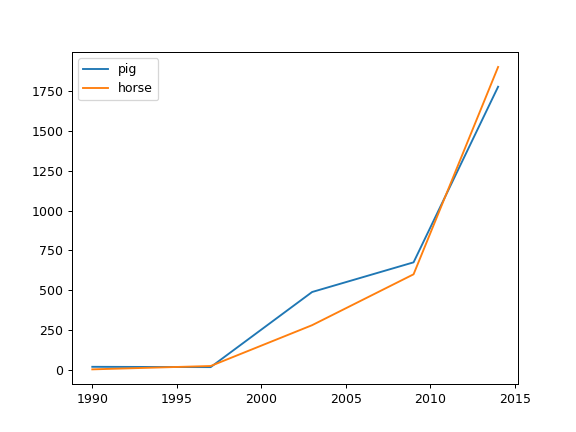An example with subplots, so an array of axes is returned.

```>>> axes = df.plot.line(subplots=True)
>>> type(axes)
<class 'numpy.ndarray'>
```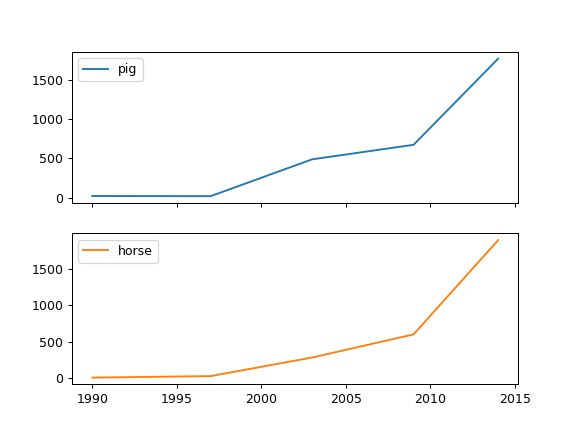Let’s repeat the same example, but specifying colors for each column (in this case, for each animal).

```>>> axes = df.plot.line(
...     subplots=True, color={"pig": "pink", "horse": "#742802"}
... )
```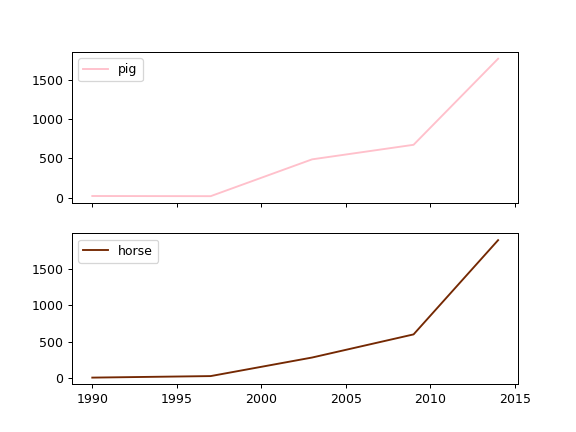The following example shows the relationship between both populations.

```>>> lines = df.plot.line(x='pig', y='horse')
```